### Home > GC > Chapter 9 > Lesson 9.2.3 > Problem9-79

9-79.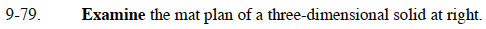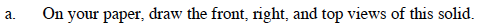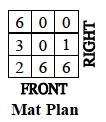Find the largest number in each column.

For each column, model that number of unit cubes stacked vertically.

Find the right view using the same technique, except going by row instead of column.

Find the top view by noting which squares have at least one unit cube.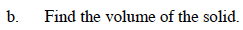The volume can easily be found by finding the sum of all the number on the mat plan, which represents the total number of unit cubes used to construct the solid.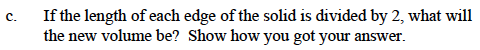Refer to the Math Notes box from Lesson 9.1.5.

3 units³

Use the eTool below to model the solid.
Click the link at right for the full version of the eTool: GC 9-79 HW eTool